# 前言

1. 了解Direct3D的一些内在规定
2. 掌握矩阵变换与2D/3D空间的联系
3. 熟悉3D变换与投影成像的过程
4. 熟悉2D变换与投影成像的过程

DirectX11 With Windows SDK完整目录

Github项目源码

# Direct3D的一些规定

## 3D坐标系

Direct3D使用的是左手坐标系，而OpenGL与我们平日接触到的数学使用的则是右手坐标系：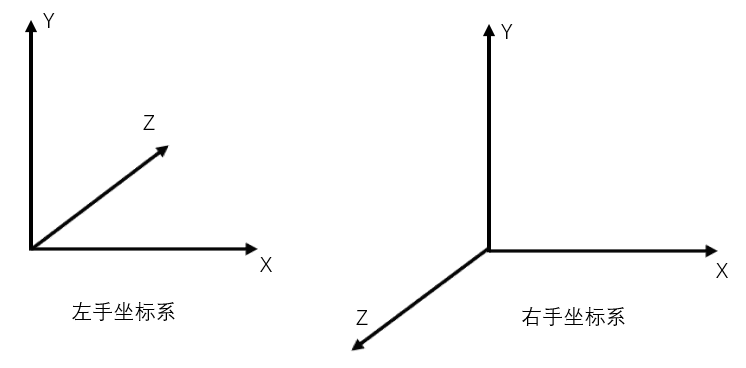## 纹理坐标系和屏幕坐标系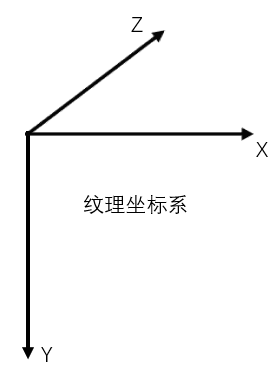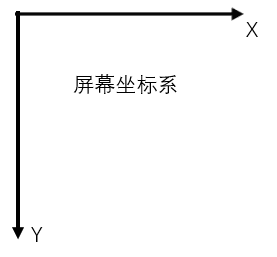## 矩阵计算

Direct3D中，矩阵通常被表示为行矩阵，即你将要使用到的DirectXMath数学库中生成的矩阵都是行矩阵。这也意味着矩阵乘法通常被表示为行向量乘以行矩阵的形式。这不仅在编写C++的代码中有所体现，在HLSL中我们也将习惯写成上述形式。

# 矩阵变换

## 变换矩阵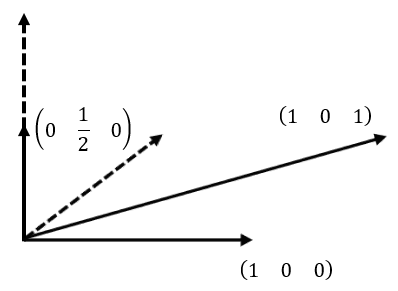$\begin{bmatrix} 2 & 4 & 1\end{bmatrix}\begin{bmatrix} 1 & 0 & 0 \\ 0 & \frac{1}{2} & 0 \\ 1 & 0 & 1 \\ \end{bmatrix}=\begin{bmatrix} 3 & 2 & 1\end{bmatrix}$

$\begin{bmatrix} x & y & z\end{bmatrix}\begin{bmatrix} \longleftarrow\vec{\mathbf{i'}}\longrightarrow \\ \longleftarrow\vec{\mathbf{j'}}\longrightarrow \\ \longleftarrow\vec{\mathbf{k'}}\longrightarrow \\ \end{bmatrix}=\begin{bmatrix} x' & y' & z'\end{bmatrix}$

## 线性变换

### 缩放

$S=\begin{bmatrix} S_x & 0 & 0 \\ 0 & S_y & 0 \\ 0 & 0 & S_z \\ \end{bmatrix}$

### 旋转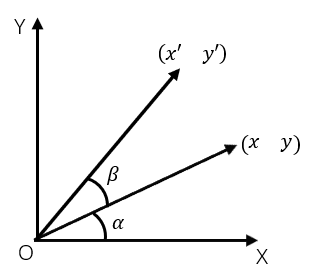$x=rcos\alpha \\ y=rsin\alpha$

$x'=rcos(\alpha + \beta) = rcos\alpha cos\beta - rsin\alpha sin\beta = xcos\beta - ysin\beta \\ y'=rsin(\alpha + \beta) = rsin\alpha cos\beta + rsin\beta cos\alpha = xsin\beta + ycos\beta$

$\begin{bmatrix} x & y \end{bmatrix}\begin{bmatrix} cos\beta & sin\beta \\ -sin\beta & cos\beta \\ \end{bmatrix}=\begin{bmatrix} x' & y' \end{bmatrix}$

$\mathbf{R_x}=\begin{bmatrix} 1 & 0 & 0 \\ 0 & cos\theta & sin\theta \\ 0 & -sin\theta & cos\theta \\ \end{bmatrix}, \mathbf{R_y}=\begin{bmatrix} cos\theta & 0 & -sin\theta \\ 0 & 1 & 0 \\ sin\theta & 0 & cos\theta \\ \end{bmatrix}, \mathbf{R_z}=\begin{bmatrix} cos\theta & sin\theta & 0 \\ -sin\theta & cos\theta & 0 \\ 0 & 0 & 1 \\ \end{bmatrix}$

$\begin{bmatrix} \frac{\sqrt{2}}{2} & \frac{\sqrt{2}}{2} & 1 & 0 \end{bmatrix}\begin{bmatrix} cos45° & sin45° & 0 & 0 \\ -sin45° & cos45° & 0 & 0 \\ 0 & 0 & 1 & 0 \\ 0 & 0 & 0 & 1 \\ \end{bmatrix}=\begin{bmatrix} 0 & 1 & 1 & 0 \end{bmatrix}$

## 仿射变换

### 齐次坐标

1. (x, y, z, 0)表示向量
2. (x, y, z, 1)表示点

1. (x, y, z, w)和(x/w, y/w, z/w, 1)都表示同一个点(w≠0)，这对于后续做透视投影会用到这个性质

### 仿射变换的定义及矩阵表示

$\begin{bmatrix} x & y & z\end{bmatrix}\begin{bmatrix} a_{11} & a_{12} & a_{13} \\ a_{21} & a_{22} & a_{23} \\ a_{31} & a_{32} & a_{33} \\ \end{bmatrix}+\begin{bmatrix} t_x & t_y & t_z\end{bmatrix}=\begin{bmatrix} x' & y' & z'\end{bmatrix}$

$\begin{bmatrix} x & y & z & 1 \end{bmatrix}\begin{bmatrix} a_{11} & a_{12} & a_{13} & 0 \\ a_{21} & a_{22} & a_{23} & 0 \\ a_{31} & a_{32} & a_{33} & 0 \\ t_x & t_y & t_z & 1 \\ \end{bmatrix}=\begin{bmatrix} x' & y' & z' & 1 \end{bmatrix}$

### 平移

$\mathbf{T}=\begin{bmatrix} 1 & 0 & 0 & 0 \\ 0 & 1 & 0 & 0 \\ 0 & 0 & 1 & 0 \\ t_x & t_y & t_z & 1 \\ \end{bmatrix}$

$\mathbf{T^{-1}}=\begin{bmatrix} 1 & 0 & 0 & 0 \\ 0 & 1 & 0 & 0 \\ 0 & 0 & 1 & 0 \\ -t_x & -t_y & -t_z & 1 \\ \end{bmatrix}$

## 变换的复合

$((v_i S)R)T={v_i}^{'}$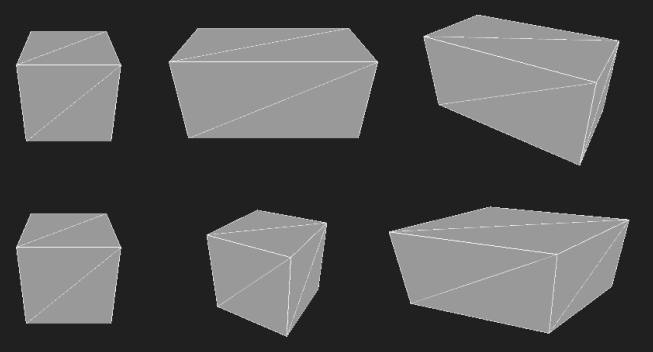## 坐标变换

$\begin{bmatrix} x & y & z & w \end{bmatrix}\begin{bmatrix} \longleftarrow\vec{\mathbf{u}}\longrightarrow & 0 \\ \longleftarrow\vec{\mathbf{v}}\longrightarrow & 0 \\ \longleftarrow\vec{\mathbf{w}}\longrightarrow & 0 \\ \longleftarrow\vec{\mathbf{Q}}\longrightarrow & 1 \\ \end{bmatrix}= \begin{bmatrix} x & y & z & w \end{bmatrix}\begin{bmatrix} u_x & u_y & u_z & 0 \\ v_x & v_y & v_z & 0 \\ w_x & w_y & w_z & 0 \\ Q_x & Q_y & Q_z & 1 \\ \end{bmatrix}= \begin{bmatrix} x' & y' & z' & w \end{bmatrix}$

# 3D的变换与投影成像

3D的部分包含了四大变换：世界变换、观察变换、投影变换和视口变换。其中前面三种变换需要在顶点着色器完成，必要时需要提供变换矩阵。而视口变换是在光栅化阶段完成的，通过第一章传给光栅化阶段的D3D11_VIEWPORT来完成。

## 局部空间与世界空间

$W=\begin{bmatrix} u_x & u_y & u_z & 0 \\ v_x & v_y & v_z & 0 \\ w_x & w_y & w_z & 0 \\ Q_x & Q_y & Q_z & 1 \\ \end{bmatrix}$## 观察空间$\mathbf{W}=\mathbf{RT}=\begin{bmatrix} u_x & u_y & u_z & 0 \\ v_x & v_y & v_z & 0 \\ w_x & w_y & w_z & 0 \\ 0 & 0 & 0 & 1 \\ \end{bmatrix}\begin{bmatrix} 1 & 0 & 0 & 0 \\ 0 & 1 & 0 & 0 \\ 0 & 0 & 1 & 0 \\ Q_x & Q_y & Q_z & 1 \\ \end{bmatrix}=\begin{bmatrix} u_x & u_y & u_z & 0 \\ v_x & v_y & v_z & 0 \\ w_x & w_y & w_z & 0 \\ Q_x & Q_y & Q_z & 1 \\ \end{bmatrix}$

$\mathbf{V}=\mathbf{W^{-1}}=\mathbf{(RT)^{-1}}=\mathbf{T^{-1}R^{-1}}=\mathbf{T^{-1}R^T}= \\ \begin{bmatrix} 1 & 0 & 0 & 0 \\ 0 & 1 & 0 & 0 \\ 0 & 0 & 1 & 0 \\ -Q_x & -Q_y & -Q_z & 1 \\ \end{bmatrix}\begin{bmatrix} u_x & v_x & w_x & 0 \\ u_y & v_y & w_y & 0 \\ u_z & v_z & w_z & 0 \\ 0 & 0 & 0 & 1 \\ \end{bmatrix}=\begin{bmatrix} u_x & v_x & w_x & 0 \\ u_y & v_y & w_y & 0 \\ u_z & v_z & w_z & 0 \\ -Q_x\cdot \mathbf{u} & Q_y\cdot \mathbf{v} & Q_z\cdot \mathbf{w} & 1 \\ \end{bmatrix}$

$\mathbf{w}=\frac{\mathbf{T-Q}}{\parallel\mathbf{T-Q}\parallel}$

$\mathbf{u}=\frac{\mathbf{j}\times\mathbf{w}}{\parallel\mathbf{j}\times\mathbf{w}\parallel}$

$\mathbf{v}=\mathbf{w}\times\mathbf{u}$DirectXMath库针对上述计算观察矩阵的处理流程提供了以下函数：

XMMATRIX XMMatrixLookAtLH(  // 输出视图变换矩阵V
FXMVECTOR EyePosition,      // 输入摄影机坐标
FXMVECTOR FocusPosition,    // 输入摄影机焦点坐标
FXMVECTOR UpDirection);     // 输入摄影机上朝向坐标


## 投影变换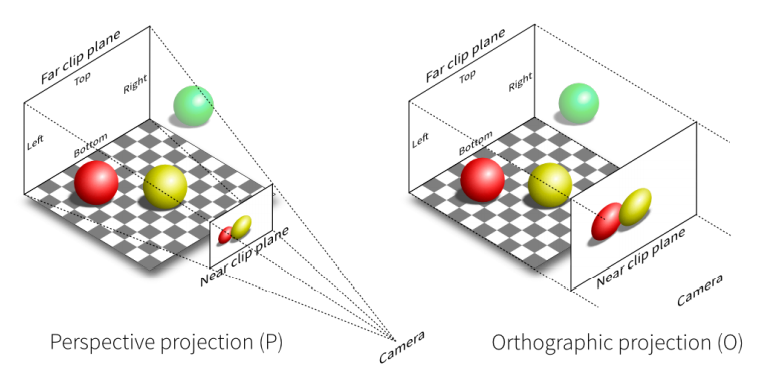## 正交投影变换与规格化设备坐标（NDC）

$-1\leq x\leq 1\\ -1\leq y\leq 1\\ 0\leq z\leq 1$

$\begin{bmatrix} \frac{w}{2} & \frac{h}{2} & n & 1 \end{bmatrix} P_{ortho} = \begin{bmatrix} 1 & 1 & 0 & 1 \end{bmatrix} \\ \begin{bmatrix} 0 & 0 & f & 1 \end{bmatrix} P_{ortho} = \begin{bmatrix} 0 & 0 & 1 & 1 \end{bmatrix} \\$

$P_{ortho}=\begin{bmatrix} \frac{2}{w} & 0 & ? & 0 \\ 0 & \frac{2}{h} & ? & 0 \\ 0 & 0 & ? & 0 \\ 0 & 0 & ? & 1 \\ \end{bmatrix}$

$\begin{bmatrix} \frac{w}{2} & \frac{h}{2} & n & 1 \end{bmatrix} \begin{bmatrix} ? \\ ? \\ ? \\ ? \end{bmatrix} = 0\\ \begin{bmatrix} 0 & 0 & f & 1 \end{bmatrix} \begin{bmatrix} ? \\ ? \\ ? \\ ? \end{bmatrix} = 1\\$

$P_{ortho}=\begin{bmatrix} \frac{2}{w} & 0 & 0 & 0 \\ 0 & \frac{2}{h} & 0 & 0 \\ 0 & 0 & A & 0 \\ 0 & 0 & B & 1 \\ \end{bmatrix}$

$nA+B=0\\ fA+B=1$

$A=\frac{1}{f-n}\\ B=\frac{-n}{f-n}$

$P_{ortho}=\begin{bmatrix} \frac{2}{w} & 0 & 0 & 0 \\ 0 & \frac{2}{h} & 0 & 0 \\ 0 & 0 & \frac{1}{f - n} & 0 \\ 0 & 0 & \frac{-n}{f - n} & 1 \\ \end{bmatrix}$

$G(z)=\frac{z}{f-n}+\frac{-n}{f-n}$

XMMATRIX XMMatrixOrthographicLH(
float ViewWidth,	// [In]待投影区域的宽度
float ViewHeight, 	// [In]待投影区域的高度
float NearZ, 		// [In]近平面
float FarZ);		// [In]远平面


$P_{ortho}=\begin{bmatrix} \frac{2}{right-left} & 0 & 0 & 0 \\ 0 & \frac{2}{top-bottom} & 0 & 0 \\ 0 & 0 & \frac{1}{f - n} & 0 \\ -\frac{right+left}{right-left} & -\frac{top+bottom}{top-bottom} & \frac{-n}{f - n} & 1 \\ \end{bmatrix}$

left和right指定投影区域的左右边界，top和bottom指定投影区域的上下边界。

XMMATRIX XMMatrixOrthographicOffCenterLH(
float ViewLeft,		// [In]待投影区域的左边界
float ViewRight, 	// [In]待投影区域的右边界
float ViewBottom, 	// [In]待投影区域的下边界
float ViewTop, 		// [In]待投影区域的上边界
float NearZ, 		// [In]近平面
float FarZ);		// [In]远平面


## 透视投影变换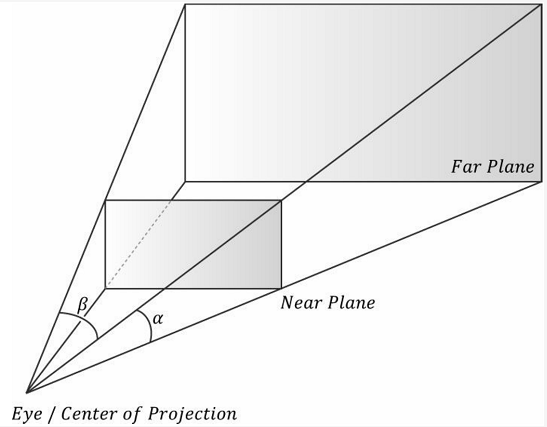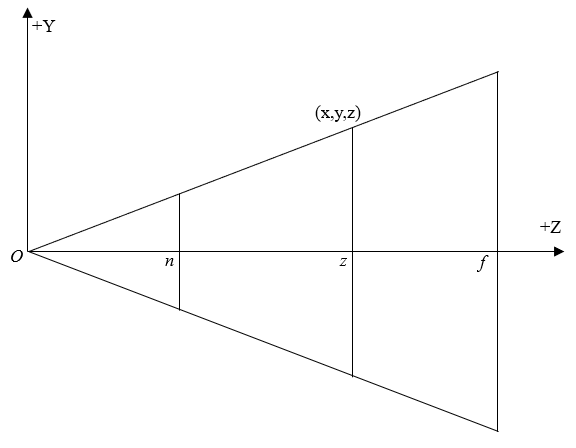### 将视锥体挤压成正交立方体

$x'=\frac{xn}{z}\\ y'=\frac{yn}{z}\\$

$\begin{bmatrix} x & y & n & 1 \end{bmatrix} P_{persp\rightarrow ortho} = \begin{bmatrix} xn & yn & n^2 & n \end{bmatrix}$

$P_{persp\rightarrow ortho} = \begin{bmatrix} n & 0 & ? & 0 \\ 0 & n & ? & 0 \\ 0 & 0 & ? & 1 \\ 0 & 0 & ? & 0 \\ \end{bmatrix}$

$\begin{bmatrix} x & y & f & 1 \end{bmatrix} P_{persp\rightarrow ortho} = \begin{bmatrix} xn & yn & f^2 & f \end{bmatrix}$

$\begin{bmatrix} x & y & n & 1 \end{bmatrix} \begin{bmatrix} ? \\ ? \\ ? \\ ? \end{bmatrix} = n^2\\ \begin{bmatrix} x & y & f & 1 \end{bmatrix} \begin{bmatrix} ? \\ ? \\ ? \\ ? \end{bmatrix} = f^2\\$

$P_{persp\rightarrow ortho} = \begin{bmatrix} n & 0 & 0 & 0 \\ 0 & n & 0 & 0 \\ 0 & 0 & A & 1 \\ 0 & 0 & B & 0 \\ \end{bmatrix}$

$nA+B=n^2\\fA+B=f^2$

$A=n+f\\B=-fn$

$P_{persp\rightarrow ortho}= \begin{bmatrix} n & 0 & 0 & 0 \\ 0 & n & 0 & 0 \\ 0 & 0 & n + f & 1 \\ 0 & 0 & -fn & 0 \\ \end{bmatrix}$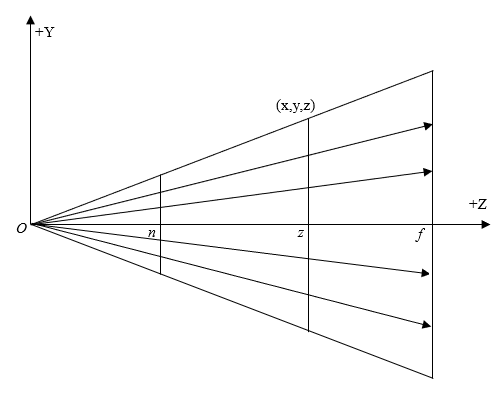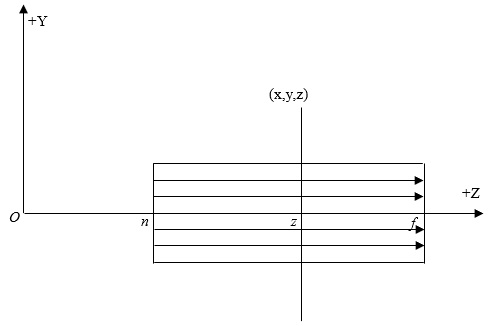$G(z)=A+\frac{B}{z}$

### 垂直视场角（FOV）和宽高比（Aspect Ratio）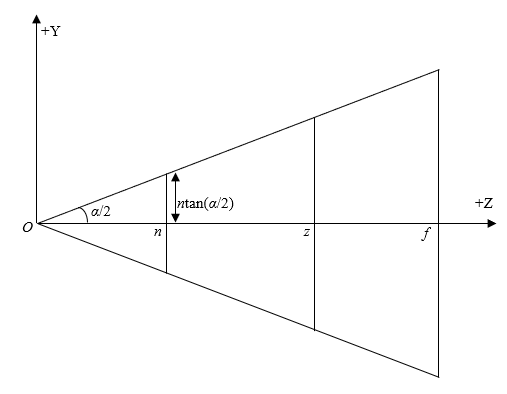$h=2n\cdot tan(\alpha/2)$

$r=w/h \\ w = 2rn\cdot tan(\alpha / 2)$

$P_{ortho}=\begin{bmatrix} \frac{1}{rn\cdot tan(\alpha/2)} & 0 & 0 & 0 \\ 0 & \frac{1}{n\cdot tan(\alpha/2)} & 0 & 0 \\ 0 & 0 & \frac{1}{f - n} & 0 \\ 0 & 0 & \frac{-n}{f - n} & 1 \\ \end{bmatrix}$

\begin{align} P_{persp}&=P_{persp\rightarrow ortho} P_{ortho} \\ &= \begin{bmatrix} n & 0 & 0 & 0 \\ 0 & n & 0 & 0 \\ 0 & 0 & n + f & 1 \\ 0 & 0 & -fn & 0 \\ \end{bmatrix}\begin{bmatrix} \frac{1}{rn\cdot tan(\alpha/2)} & 0 & 0 & 0 \\ 0 & \frac{1}{n\cdot tan(\alpha/2)} & 0 & 0 \\ 0 & 0 & \frac{1}{f - n} & 0 \\ 0 & 0 & \frac{-n}{f - n} & 1 \\ \end{bmatrix} \\ &= \begin{bmatrix} \frac{1}{rtan(\alpha/2)} & 0 & 0 & 0 \\ 0 & \frac{1}{tan(\alpha/2)} & 0 & 0 \\ 0 & 0 & \frac{f}{f-n} & 1 \\ 0 & 0 & -\frac{nf}{f-n} & 0 \\ \end{bmatrix} \end{align}

### 归一化深度值

$g(z)=\frac{f}{f-n}-\frac{nf}{(f-n)z}$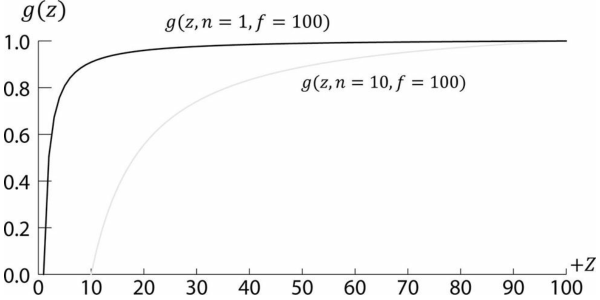XMMATRIX XMMatrixPerspectiveFovLH( // 返回投影矩阵
FLOAT FovAngleY,                   // 中心垂直弧度
FLOAT AspectRatio,                 // 宽高比
FLOAT NearZ,                       // 近平面距离
FLOAT FarZ);                       // 远平面距离


# 2D的变换与投影成像

Direct3D不仅能够绘制3D物体，还可以在后备缓冲区直接绘制2D平面物体。当然，也可以使用Direct2D来绘制2D物体（后续章节会涉及到Direct2D与Direct3D的交互）。

## 2D局部空间与2D世界空间

2D世界变换也囊括了缩放、旋转和平移变换。通过世界变换，我们可以将物体模型从自身的局部坐标系转换到世界坐标系中。当然，我们也可以理解为将物体从世界原点开始缩放、旋转，然后平移到目标位置。这样每一个物体都能在同一个世界空间中表示。

$W=\begin{bmatrix} u_x & u_y & 0 & 0 \\ v_x & v_y & 0 & 0 \\ 0 & 0 & 1 & 0 \\ Q_x & Q_y & 0 & 1 \\ \end{bmatrix}$

## 正交投影变换与规格化设备坐标（NDC）

$-1\leq x\leq 1\\ -1\leq y\leq 1\\ 0\leq z\leq 1$

# HLSL变量命名的一些约定

L 处于物体局部空间（Local Space）
W 处于世界空间（World Space）
V 处于观察空间（View Space）
H 处于齐次裁减空间（Homogeneous space）

# 练习题

1. 若有兴趣，可以去了解一下绕任意轴旋转的矩阵推导过程。本章不列出。
2. 若有兴趣，可以去了解一下透视校正插值法。本章不列出。
3. 修改项目03，在窗口被拉伸的情况下修改透视投影矩阵的宽高比保证物体正常显示。
4. 修改项目03，观察透视投影矩阵下不同FOV值的效果，以及将远平面设置为5.0f的效果
5. 修改项目03，让摄像机位于点(1, 0, -2)观察原点，并使用正交投影观察效果
6. 修改项目03，让立方体中心位于点(3, 0, 0)，然后立方体按Z轴绕原点逆时针旋转，同时它也按Z轴绕立方体中心顺时针旋转，速度自拟。

DirectX11 With Windows SDK完整目录

Github项目源码

posted @ 2017-07-26 20:54  X_Jun  阅读(5547)  评论(0编辑  收藏  举报
levels of contents